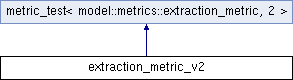extraction_metric_v2 Struct Reference

`#include <extraction_metrics_test.h>`

Inheritance diagram for extraction_metric_v2:## Static Public Member Functions

static void create_expected (metric_set_t &metrics, const model::run::info &=model::run::info())

template<class Collection >
static void create_binary_data (Collection &buffer)

static void create_summary (model::summary::run_summary &summary)Static Public Member Functions inherited from metric_test< model::metrics::extraction_metric, 2 >
static std::string name ()

static std::vector< T > to_vector (const T(&vals)[N])

static std::vector< T > to_vector (const sparse_value< T, Size >(&vals)[N])

## Additional Inherited MembersPublic Types inherited from metric_test< model::metrics::extraction_metric, 2 >
enum  Checks

typedef model::metrics::extraction_metric metric_t

typedef model::metric_base::metric_set< model::metrics::extraction_metricmetric_set_t

typedef metric_set_t::const_iterator const_iterator

## Detailed Description

This generator creates an expected metric set and the corresponding binary data

model::metrics::extraction_metric
Note
Version 2

## Member Function Documentation

 static void create_binary_data ( Collection & buffer )
inlinestatic

Get the expected binary data

Parameters
 buffer binary data string
 static void create_expected ( metric_set_t & metrics, const model::run::info & = `model::run::info()` )
inlinestatic

Create the expected metric set

Parameters
 metrics destination metric set
 static void create_summary ( model::summary::run_summary & summary )
inlinestatic

Get the summary for these metrics

Returns
run summary

The documentation for this struct was generated from the following file: